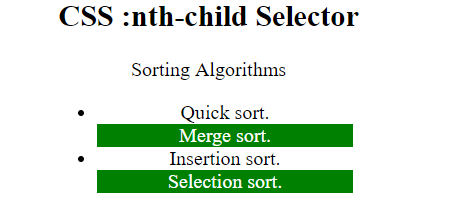Open in App
Not now

# CSS :nth-child() Selector

• Last Updated : 22 Aug, 2022

The :nth-child() CSS pseudo-class selector is used to match the elements based on their position in a group of siblings. It matches every element that is the nth-child, regardless of the type, of its parent.

Syntax:

```:nth-child(number) {
// CSS Property
}```

Where number is the single argument that represents the pattern for matching elements. It can be odd, even, or in a functional notation.

• odd: It represents elements whose position is odd in a series: 1, 3, 5, etc.
• even: It represents the elements whose position is even in a series: 2, 4, 6, etc.
• functional notation (<An+B>): It represents elements whose position of siblings matches the pattern An+B, for every positive integer or zero value of n. Here, A represents the integer step size, B represents the integer offset.

Example 1: In this example, every odd paragraph is selected. Formula used is 2n+1 i.e 1, 3, 5, etc paragraphs are selected.

## HTML

 `` `<``html``>`   `<``head``>` `    ``<``title``>CSS :nth-child Selector` `    ``<``style``>` `    ``p:nth-child(2n+1) {` `        ``background: green;` `        ``color: white;` `    ``}` `    ``` ``   `<``body` `style``=``"text-align:center"``>` `    ``<``h1` `style``=``"color:green;"``>` `            ``GeeksforGeeks` `        ``` `    ``<``h2``>` `            ``CSS :nth-child Selector` `        ``` `    `  `<``p``>A computer science portal for geeks.`     `    `  `<``p``>Geeks classes an extensive classroom programme.`     ``   ``

Output:Example 2: In this example, every even <li> is selected i.e. 2, 4, 6, etc.

## HTML

 `` `<``html``>`   `<``head``>` `    ``<``title``>CSS :nth-child Selector` `    ``<``style``>` `    ``li {` `        ``width: 30%;` `    ``}` `    `  `    ``li:nth-child(even) {` `        ``background: green;` `        ``color: white;` `    ``}` `    ``` ``   `<``body` `style``=``"text-align:center"``>` `    ``<``h2``>` `            ``CSS :nth-child Selector` `        ``` `    `    `<``p``>Sorting Algorithms`     `    ``<``ul``>` `        ``<``li``>Quick sort.` `        ``<``li``>Merge sort.` `        ``<``li``>Insertion sort.` `        ``<``li``>Selection sort.` `    ``` ``   ``

Output:Supported Browsers: The browser supported by :nth-child() selector are listed below: